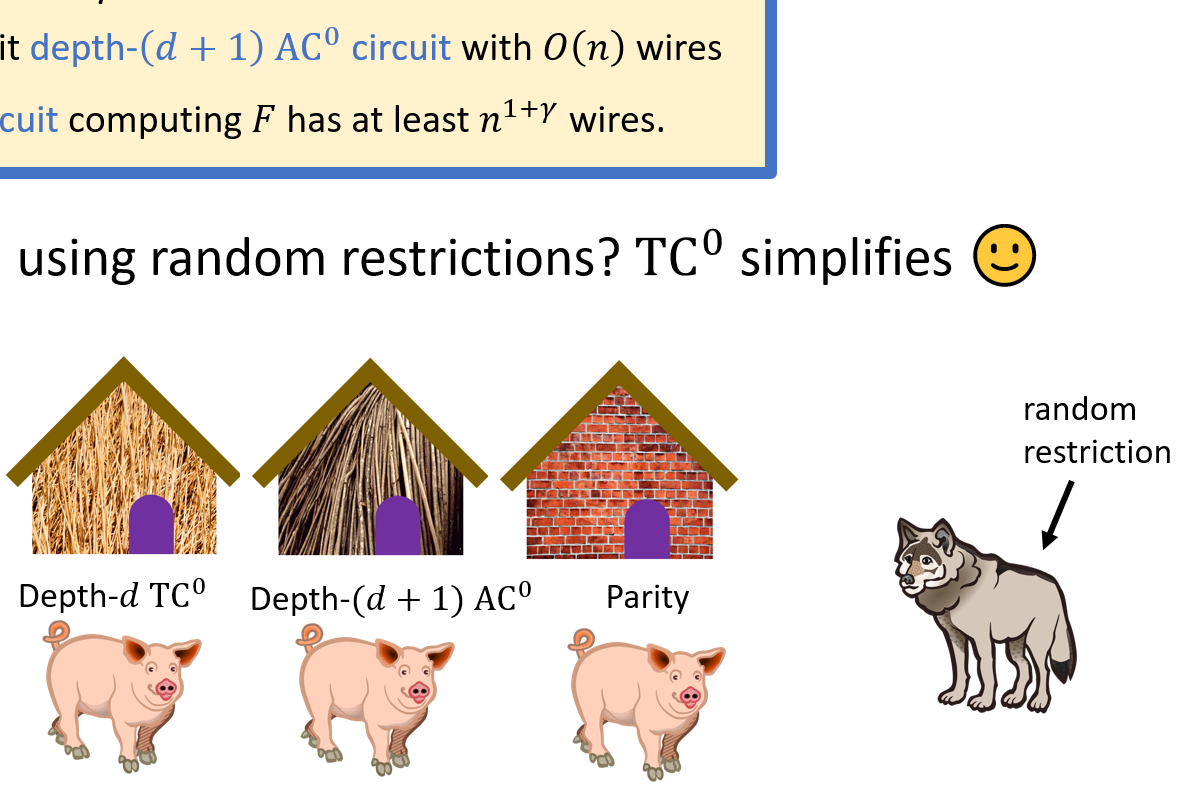Back to list of papers

# Depth-$$d$$ Threshold Circuits vs. Depth-$$(d + 1)$$ AND-OR Trees

By Pooya Hatami, William M. Hoza, Avishay Tal, and Roei Tell

Abstract (for specialists)

For any $$n \in \mathbb{N}$$ and $$d = o(\log \log n)$$, we prove that there is a Boolean function $$F$$ on $$n$$ bits and a value $$\gamma = 2^{-\Theta(d)}$$ such that $$F$$ can be computed by a uniform depth-$$(d + 1)$$ $$\mathsf{AC}^0$$ circuit with $$O(n)$$ wires, but $$F$$ cannot be computed by any depth-$$d$$ $$\mathsf{TC}^0$$ circuit with $$n^{1 + \gamma}$$ wires. This bound matches the current state-of-the-art lower bounds for computing explicit functions by threshold circuits of depth $$d > 2$$, which were previously known only for functions outside $$\mathsf{AC}^0$$ such as the parity function. Furthermore, in our result, the $$\mathsf{AC}^0$$ circuit computing $$F$$ is a monotone read-once formula (i.e., an $${\mathsf{AND}\text{-}\mathsf{OR}}$$ tree), and the lower bound holds even in the average-case setting with respect to advantage $$n^{-\gamma}$$.

At a high level, our proof strategy combines two prominent approaches in circuit complexity from the last decade: The celebrated random projections method of Håstad, Rossman, Servedio, and Tan (J. ACM 2017), which was previously used to show a tight average-case depth hierarchy for $$\mathsf{AC}^0$$; and the line of works analyzing the effect of random restrictions on threshold circuits. We show that under a modified version of Håstad, Rossman, Servedio, and Tan's projection procedure, any depth-$$d$$ threshold circuit with $$n^{1 + \gamma}$$ wires simplifies to a near-trivial function, whereas an appropriately parameterized $${\mathsf{AND}\text{-}\mathsf{OR}}$$ tree of depth $$d + 1$$ maintains structure.

Not-so-abstract (for curious outsiders)

⚠️ This summary might gloss over some important details.

A "Boolean circuit" is an idealized network of logic gates and input variables connected by wires. You can assign binary values to the input variables. The values feed into whatever gates are connected to the variables. The outputs of those gates feed into other gates, which feed into other gates, and so on, until eventually the circuit produces a binary output.

In one basic circuit model, the only available logic gates are "AND" gates and "OR" gates. (An "AND" gate outputs 1 if its inputs are all 1. An "OR" gate outputs 1 if at least one of its inputs is 1.) There is also a more powerful circuit model in which we allow "threshold gates," which compute an arbitrary weighted sum of their input bits and output 1 if the weighted sum is above some threshold. This second type of circuit can be considered a simplified model of a brain.

What are threshold gates good for? How much additional power do they give you compared to AND/OR gates? It has been known for decades that using threshold gates, it is possible to decrease the depth of a given AND/OR circuit. (The "depth" of a circuit is the length of the longest path from input to output, which can be considered a measure of the amount of time that the computation takes.)

The caveat is that known depth-reduction methods substantially increase the total number of wires in the circuit, which is a measure of the total amount of work that goes into the computation. In our paper, we prove that increasing the number of wires is unavoidable in general. That is, we prove that decreasing the depth of certain AND/OR circuits using threshold gates requires increasing the number of wires by a non-negligible amount.

We posted a manuscript online in June 2022; I presented the paper at STOC in June 2023. The STOC proceedings version is merely an "extended abstract" with no proofs.

Expository material:Slides from my presentation at Dagstuhl Seminar 22371 (September 2022). See also these shorter slides from my presentation at STOC (June 2023), or these other short slides from my presentation at TOCA-SV (May 2022), or this poster that I presented at TOCA-SV the same day.

A prerecorded video made by Roei for STOC (June 2023).